# 1st PUC Chemistry Question Bank Chapter 6 Thermodynamics

Students can Download Chemistry Chapter 6 Thermodynamics Questions and Answers, Notes Pdf, 1st PUC Chemistry Question Bank with Answers helps you to revise the complete Karnataka State Board Syllabus and to clear all their doubts, score well in final exams

## Karnataka 1st PUC Chemistry Question Bank Chapter 6 Thermodynamics

Question 1.
What is thermodynamics?
The study of interconversion of heat and other kinds of energy is called thermodynamics.

Thermodynamic Terms

Question 1.
Define fundamental terms in thermodynamics.
(a) System: A part of universe under study or consideration is called the system.

(b) Surroundings: The remaining part of the universe that can interact with the system is called surroundings.
Note: System and the surroundings together constitute the universe. Universe = System + Surroundings

(c) Open system: A system which can exchange both matter and energy with its surroundings is called open system. Example: Hot water in a open vessel.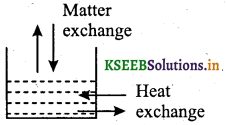(d) Closed system: A system which can exchange only energy but not matter with its surroundings is called a closed system. Example: Pressure cooker.(e) Isolated system: A system which can exchange neither energy nor matter with its surrounding is called an isolated system. Example: Hot coffee kept in a thermoflask.(f) State of a system: A system is said to be in a given state, when the thermodynamic variables like pressure, volume, temperature and composition of the system have fixed value.

(g) State function: A function which depends only on the initial state and final state of the system but not on the path is called state function. Example: Internal energy, entropy, enthalpy etc.

Note: Thermodynamic variables such as pressure, temperature, volume and composition are called state functions because the change in their values depends only on the initial and final states of the system.Question 2.
Define internal energy.
The internal energy is the total amount of kinetic and potential energy possessed by the system. It is denoted by the symbol U. The internal energy is made up of different kinds of energies like translational energy of molecules (Ut), rotational energy of molecules (Ur), vibrational energy of molecules (Uv), electronic energy (Ue), nuclear energy (Un), and gravitational energy (Ug),
U = Ut + Ur + Uv + Ue + Un + Ug
Internal energy of any substance depends on temperature and amount of the sample.

Question 3.
Internal energy is a state function. Explain.
Internal energy is a state function, i.e., its value depends only upon the state of the substance but does not depend upon how that state is achieved.
The absolute value of internal energy of a substance cannot be determined. But change in internal energy can be measured experimentally.
ΔU = Ufinal – Uinitial
ΔUreaction = Uproducts – Ureactants
ΔU = U2 – U1, where U1 is initial internal energy and U2 final internal energy.
Note: Internal energy of the system, will change, when

• Heat passes in or out of the system,
• Work is done on or by the system,
• Matter enters or leaves the system.

Question 4.
A process in which there is no exchange of heat between the system and surrounding is known as adiabatic process. In an adiabatic process there is a change in temperature of the system but there is no heat exchange between the system and surroundings. Therefore ΔT ≠ 0 and q = 0.

Question 5.
State first law of thermodynamics. Write its mathematical form.

• The first law of thermodynamics states that “the energy of an isolated system is constant”.
• “energy can neither be created nor be destroyed, but it can be transformed from one form to another”.
The mathematical form of first law of thermodynamics is
ΔU = q + W

Question 6.
Express the change in internal energy of a system when
(i) No heat is absorbed by the system from the surroundings, but work (w) is done on the system. What type of wall does the system have?
(ii) No work is done on the system, but q amount of heat is taken out from the system and given to the surroundings. What type of wall does the system have?
(iii) w amount of work is done by the system and q amount of heat is supplied to the system. What type of system would it be?
(ii) ΔU = – q, thermally conducting walls
(iii) ΔU = q – w, closed system.

Question 7.
What is isothermal process?
A process takes place at constant temperature is called isothermal process.

Question 8.
What is reversible process?
A process which is carried out infinitesimally slowly, such that it can be reversed at any instant of time by reversing the driving force by infinitesimally amount is called a reversible process.

Question 9.
What is irreversible process?
A rapid process which cannot be reversed at any instant of time by reversing the driving force by a small amount is called irreversible process.Applications

Question 1.
Explain different types of energy changes in thermodynamic systems.
Energy charges in thermodynamic process takes place in the form of work and heat.
(a) Work: Exchange of energy between system and surroundings can occur in the form of work which may be mechanical or electrical work
(b) Heat: The exchange of energy as a result of temperature difference is called heat (q).
∴ q α ΔT.
In this case change in internal energy, ΔU = q, when no work is done at constant volume.
q is positive, when heat is transferred from the surroundings to the system and q is negative when heat is transferred from system to the surroundings.

Question 2.
Derive an expression for the mechanical work done in an isothermal irreversible compression of an ideal gas.
Let us consider a cylinder which contains one mole of an ideal gas fitted with a frictionless movable piston.
Let Vi, be the total volume of the gas and p be the pressure of the gas inside.
Let ‘Vi’ be the initial volume of the gas. If an external pressure is applied on the piston which is greater than the internal pressure, piston moves inward till the pressure inside is equal to external pressure. The volume of the gas is decreased and final volume is ‘Vf’ During this compression, the piston moves through a distance ‘l’ then,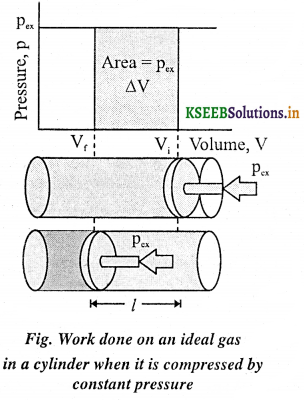Volume change = l A = ΔV = Vf – Vi
But, pressure = Force/area
Therefore, force on the piston = pressure × area
= Pext. A

If ‘w’ is the work done on the system by the movement of the piston, then
w = force × distance
= PextA l
= Pext. (-ΔV)
= -Pext.(ΔV)
w = -Pext.(Vf – Vi)

Note: ‘w’ becomes positive when work is done on the system during compression. Here, Vf – Vi becomes negative because Vf < Vi during compression.
If the pressure is not constant at every stage of compression, the work is equal to sum of all the steps:
Therefore, w = -Σ pext. ΔVQuestion 3.
Derive an expression for the mechanical work done in an isothermal reversible expansion of an ideal gas.
During reversible isothermal expansion of an ideal gas, the gas expands in a large number of steps through small volumes against the external pressure [pext].
Suppose the gas expands through a small volume ‘dV’ against external pressure, pext, the small work done by the gas is given by .
δw = -pext . dV
For reversible expansion, pext = pint – dp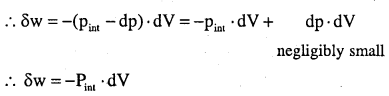Total work done by the gas from an initial volume of V, to final volume of V2 is got by integrating the above equation.Question 4.
What is free expansion? What is the work done during the expansion of an ideal gas both in reversible and irreversible process?
Expansion of a gas in vacuum (pext = 0) is called free expansion.
No work done (w = 0) during free expansion of an ideal gas whether the process is reversible or irreversible.

Question 5.
Write the expression for work done in irreversible isothermal free expansion of ideal gas.
ΔU = q + w
In isothermal process, ΔU = 0
∴ q = -w
∴ q = -(-pext(vf – vi))
q = Pext(Vf – Vi)Question 6.
Write the expression in reversible isothermal free expansion of ideal gas.
ΔU = q + w
In isothermal process
ΔU = 0
∴ q = -w
q = -(-2.303 nRT log $$\frac{V_{f}}{V_{i}}$$)
q = +2.303 nRT log $$\frac{V_{f}}{V_{i}}$$
Note: For adiabatic change, q = 0

Numerical Problems

Question 1.
What is the change in internal energy of a system, if 20 J of heat is supplied to it and 25 J of work is done by it?
ΔU = q + w
= 20 – 25
= -5 J

Question 2.
A gas absorbs 120 J of heat and expands against the external pressure of 1.10 atm from a volume of 0.5 L to 2.0 L. What is the change in internal energy? (1 L atm = 101.3 J)
ΔU = q + w
q = +120 J
w = -pext(v2 – v1)
= -1 . 10 × 1 . 5 = -1 . 65 Latm
= -1.65 × 101.3 = -167.145 J
∴ ΔU = (+120) + (-167.145)
= -47.135 JQuestion 3.
Calculate the work done when a gas expands at a constant temperature from volume 2 × 10-3 m3 to 4 × 10-3 m3 against a constant pressure of 1.2 × 105 Nm-2.
w = -pΔV
p = 1.2 × 105Nm-2; ΔV = Vf – Vj =(4 × 103) – (2 × 103) = 2 × 10-3m3; w = ?
w= -1.2 × 105Nm-2 × 2 × 10-3m3 = -2-4 × 102 Nm
= -2-4 × 102J (since Nm = J)

Question 4.
Calculate the total work done when 1 mole of a gas expands isothermally and reversity from an initial value of 20 dm3 to a final value of 40 dm3 at 298 K (R = 8.314 JK-1 mol-1).
w = -2.303 nRT log $$\frac{V_{2}}{V_{1}}$$
n = 1, R = 8.314 JK-1mol-1, T = 298 K.V1 =20dm3, V2 = 40dm3,w = ?
w = -2.303 × 8.314 × 298 log $$\frac { 40 }{ 20 }$$
= -2.303 × 8.314 × 298 log2
= -2.303 × 8.314 × 298 × 0.3010 = -1717.46 Jmor-1

Question 5.
Two moles of an ideal gas initially at 27°C and one atmospheric pressure are compressed isothermally and reversibility till the final pressure of the gas is 10 atm. Calculate q, w and ΔU for the process.
Here, n = 2 moles, T = 27°C = 300 K, p1 =1 atm, p2 = 10 atm
w = 2 . 303 nRT log$$\frac{p_{2}}{p_{1}}$$
= 2.303 × 2 × 8.314 × 300 × log$$\frac{10}{1}$$
= 2.303 × 2 × 8.314 × 300 × 1 = 11488.3 J
For isothermal compression of ideal gas, ΔU = 0
Further, ΔU = q + w ∴ q = -w = -11488.3 J

Question 6.
Two litres of an ideal gas at a pressure of 10 atm expands isothermally into vacuum until its total volume is 10 litres. How much heat is absorbed and how much work is done in the expansion? What would be the heat absorbed and work done, (i) If the same expansion takes place against a constant external pressure of 1 atm? (ii) If the same expansion takes place to a final volume of 10 litres conducted reversibly?
Work of expansion against vacuum is zero. ∴ w = 0
For an isothermal change, ΔU = 0
ΔU = q + w ∴ q = 0
∴ q = -w = Pex(10L – 2L) = 0(8) = 0

(i) q = -w = 1 atm × 8L = 8Latm
= 8 × 101.3 J = 810.4 J (1 L atm = 101.3 J)

(ii) q = -w = 2.303 RTlog$$\frac{V_{2}}{V_{1}}$$ (v pV = RT)
= 2.303 pV log $$\frac{V_{2}}{V_{1}}$$
= 2.303 × 10 atm × 2Llog$$\frac{10}{3}$$ = 32.2 L atm
= 32.2 × 101.3 = 3261.86JQuestion 7.
Calculate the amount of work done in each of the following cases: (i) One mole of an ideal gas contained in a bulb of 10 litre capacity at 1 bar allowed to enter into an evacuated bulb of 100 litre capacity, (ii) One mole of a gas is allowed to expand from a volume of 1 litre to a volume of 5 litres against the constant external pressure of 1 bar (1 litre bar = 100 J). Calculate the internal energy change (ΔU) in each case if the process were carried out adiabatically.
(i) w = -pext × ΔV
As expansion takes place into the evacuated bulb, i.e., against vacuum, pext = 0. Hence, w = 0.
For adiabatic process, q = 0 ∴ ΔU = q + w = 0 + 0 = 0

(ii) ΔV = Vf – Vj = 5 – 1 = 4 L, p = l bar
w = -pΔV
= -1 × 4 = -4Lbar = -4 × 100 J = -400 J
The negative sign implies that the work is done by the system.
For adiabatic process, q = 0. Hence, ΔU = q + w = 0 – 405 . 2 J = -405 . 2 J

Question 8.
If a balloon is inflated from 0.100 L to 1.85 L against an external pressure of 1.00 atm, how much work is done? .
Given: Vi = 0.100 L, Vf = 1.85 L, p = 1.00 atm.
W = -PextΔV
ΔV = Vf – Vi = 1.85 L – 0.10 L =1.75 L.
w = – (1 atm) (1.75 L) = – 1.75 atm L.
w (joule) =-1.75 × 101.3 J =-177 J

Question 9.
Define enthalpy (H).
It is defined as the sum of the internal energy and the product of pressure and volume.
H = U + pV
Note: The enthalpy change (ΔH) of a reaction is the heat evolved in a reaction at constant pressure.
ΔH = qp

Question 10.
Enthalpy is a state function. Explain.
Enthalpy is a state function because it depends only on the initial and final state of the system but not on the path.

Question 11.
Write the relation between enthalpy change and internal energy change.
ΔH = ΔU + pΔV

Question 12.
What is exothermic reaction?
A reaction in which heat is evolved or liberated is called exothermic reaction.
C(s) + O2(g) → CO2(g); ΔH = -393.5 kJ

Question 13.
What is endothermic reaction?
A reaction during which heat is absorbed is called endothermic reaction.
N2(g) + O2(g) → 2NO(g) ; ΔH = +180.4 kJQuestion 14.
Difference between ΔH and ΔU is not significant for systems containing only solids and liquids. Why?
Because solids and liquids do not suffer any significant volume changes upon heating.

Question 15.
Difference between ΔH and ΔU is significant for system containing gases. Why?
Because there is significant volume change in gases on heating.

Question 16.
Derive the relation between ΔH and ΔU for the system containing gases, or Derive the relation ΔH = ΔU + ΔngRT.
Let us consider a reaction involving gases. If VA is the total volume of the gaseous reactants, VB is the total volume of the gaseous products, nA is the number of moles of gaseous reactants and nB is the number of moles of gaseous products, all at constant pressure and temperature, then using the ideal gas law, we write,
PVA = nART
and pVB = nBRT
Thus, pVB – pVA = nBRT – nART = (nB – nA)RT
or P(VB – VA) = (nB – nA )RT
or pΔV = ΔngRT
Here, Δng refers to the number of moles of gaseous products minus the number of moles of gaseous reactants. Substituting the value of pΔV in the equation ΔH = ΔU + pΔV we get
ΔH = ΔU + ΔngRTNumerical Problems

Question 1.
The reaction of cyanamide, NH2CN(s) with oxygen was determined in a bomb calorimeter and AU was found to be -742.7 kJ mol-1 of cyanamide at 298 K. Calculate the enthalpy change for the reaction
NH2CN(S) + $$\frac { 3 }{ 2 }$$ O2(g) →N2(g) + CO2(g) + H2O(l) at 298 K.
Δng = nP(g) – nR(g)
Δng = 2 – $$\frac { 3 }{ 2 }$$ = + 0.5
R = 8.314 × 10-3 kJK-1 mol-1; T = 298 K ; ΔU =-742.7 kJ mol-1
ΔH = ΔU + Δng RT
ΔH = -742.7(kJ) + 0.5(mol) × 8.314 × 10-3 (kJ K-1 mol-1) × 298 (K)
= -742.7 kJ + 1.238.78 kJ = -741.46kJ mol-1

Question 2.
Calculate the difference in heat of reaction at constant pressure and constant volume for the reaction
2C6H6(1) + 15O2(g) → 12CO2(g) + 6H2O(1) at 25°C.
2C6H6(1) + 15O2(g) → 12CO2(g) + 6H2O(1)
ΔH = ΔU + ΔngRT
Δng = np(g) – nR(g)
= 12 – 15 = – 3
R = 8.314JK-1 mol-1 ⇒ 8.314 × 10-3 kJ K-1mol-1
T = 25°C + 273 = 298K
ΔH – ΔU = -3 × 8.314 × 298 × 10-3
= -7.433kJ

Question 3.
Calculate the standard change in internal energy at 300 K for the reaction
CCl4(I) + 2H2O(g) → CO2(g) + 4HCl(g) when standard enthalpies of formation for CCl4(1), H2O(g), CO2(g) and HCl(g) are – 107, -242, -394 and -93 kJ mol-1.
Given, CCl4(1) + 2H2O(g) → CO2(g) + 4HCl(g)
ΔHreaction = ΣHProducts – ΣHreactants
ΔHreaction = ΔfH(CO2)(g) + 4ΔfH(HCl)(g) – ΔfH(CCl4)(l) – 2ΔfH(H2O)1(g)
= [-394 + (4 × -93)] – (-107) – (2 × -242)]
= -394 – 372 +107 + 484 = – 175 kJ mol-1.
ΔH = ΔU + ΔngRT
ΔU = ΔH – Δng RT
Δn = np (g) – nR (g) = 5 – 2 = 3
∴ ΔU = -175kJmol-1 -3(8.314 × 10-3 kJ K-1 mol-1)(300K)
= -182.5 kJ mol-1Question 4.
If water vapour is assumed to be a perfect gas, molar enthalpy change for vaporisation of 1 mol of water at 1 bar and 100 °C is 41 kJ mol-1. Calculate the internal energy change, when (i) 1 mol of water is vaporised at 1 bar pressure and 100 °C (ii) 1 mol of water is converted into ice.
(i) The change H2O(l) → H2O(g)
Δng = 1 – 0 = 1
ΔH = ΔU + Δng RT
or ΔU = ΔH – ΔngRT, substituting the values, we get
ΔU = 41.00 kJmol-1 – 1 × 8.314 × 10-3kJ mol-1 K-1 × 373 K
= 41.00kJ mol-1 – 3.101 kJ mol-1 = 37.808 kJ mol-1

(ii) The change H2O(l) → H2O(s)
There is negligible change in volume,
So, we can put = ΔngRT = 0. ∴ ΔH = ΔU
So, ΔU = 41.00 kJmol-1

Question 5.
A swimmer coming out from a pool is covered with a film of water weighing about 18 g. How much heat must be supplied to evaporate this water at 298 K? Calculate the internal energy of vapourisation at 100 ° C. ΔvapH° for water at 373 K = 40.66 kj mol-1
The process of evaporation may be represented as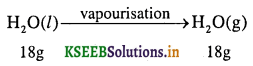Number of moles in 18 g H2O(l)is = $$\frac{18 \mathrm{g}}{18 \mathrm{g} \mathrm{mol}^{-1}}$$ = lmol
18gmol
ΔvapU = ΔvapH°- ΔngRT
= 40.66 × 103 Jmol-1 – (1)(8.314 JK-1 mol-1)(373K)
= 40660 – 3101 . 122 = 37558.878 J mol-1 = 37.558 kJ mol-1

Question 6.
What is extensive property? Give examples.
A property which depends of the quantity of matter present in the system is called extensive property. Examples’. Mass, volume, internal energy, heat, free energy, entropy, etc.Question 7.
What is Intensive property? Give examples.
A property which is independent on the quantity of matter present in the system is called intensive property. Examples: Density, surface tension, viscosity, specific heat, thermal conductivity, refractive index, pressure, temperature, boiling point, freezing point, vapour pressure of a liquid, etc.

Question 8.
Define heat capacity.
Heat capacity (C) is the amount of heat required to change the temperature of substance by a given amount.

Question 9.
Define molar heat capacity.
The amount of heat required to rise the temperature of one mole of a substance by one degree Celsius or one Kelvin is called molar heat capacity (cm).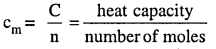Question 10.
Define specific heat capacity.
The amount of heat required to rise the temperature of unit mass of a substance by one degree Celsius or one Kelvin is called specific heat capacity.
To find the heat required to increase the temperature of any substance, the following formula is used.
Heat absorbed = Specific heat x Mass of the substance x Temperature change
q = c × m × ΔT
But, c × m = C
q = C × ΔT

Question 11.
Derive the relation between Cp and Cv for gas. or Derive Cp – Cv = R.
We know that, q = C × ΔT …(1)
At constant volume, qv = Cv × ΔT = ΔU …(2)
At constant pressure qp = Cp × ΔT = ΔH …(3)
For one mole of an ideal gas, ΔH = ΔU + Δ(RT)
CpΔT = CvΔT + Δ(RT)
CpΔT = Cv ΔT + RΔT
Cp = Cv + R
Cp – Cv = RMeasurement of ΔU and ΔH: Calorimetry

Question 1.
Describe the experiment to determine the internal energy change by bomb calorimeter with neat labeled diagram.
A convenient device for the measurement of internal energy change is bomb calorimeter. A “bomb” calorimeter is an apparatus designed to measure the heat given off during combustion reactions at constant volume. The reaction takes place in a heavy- duty stainless steel cylinder called a “bomb.” The “bomb” has a threaded lid so that it can be sealed (like screwing two pieces of metal pipe together). A small length of ignition wire is inserted through which an electric current is passed rapidly thereby initiating the combustion. In the bomb, weighed sample of a combustible substance is placed together with o2 (g) at about 30 atm pressure. The bomb is in contact with a water-bath which is constantly stirred to help ensure a uniform temperature throughout the water-bath. When the substance is ignited by passing electrical current through the heating wire, the heat energy released by its combustion raises the temperature of water surrounding the bomb. Measurement of the change in temperature of the water permits calculation of ΔU.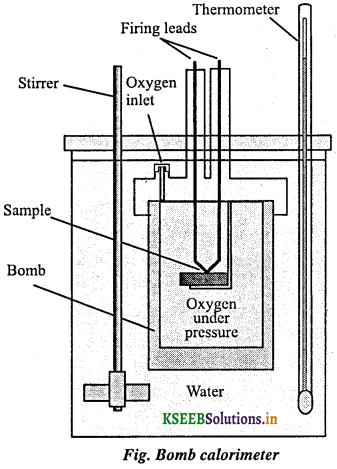Question 2.
Describe the experiment to determine the enthalpy change of a reaction (heat of reaction at constant pressure, ΔH) by calorimeter.
The enthalpy change of a reaction is measured in a calorimeter. The calorimeter is kept open to the atmosphere. The calorimeter is immersed in an insulated water-bath fitted with stirrer and thermometer. The temperature of the water-bath is recorded in the beginning and after the end of the reaction. The change in temperature is calculated. Knowing the heat capacity of water-bath and calorimeter and also the change in temperature, the heat absorbed or evolved in the reaction is calculated. This gives the enthalpy change (ΔH) of the reaction. It is calculated by the formula,
ΔH = Specific heat × Mass of substance × Temperature change
= c × m × ΔT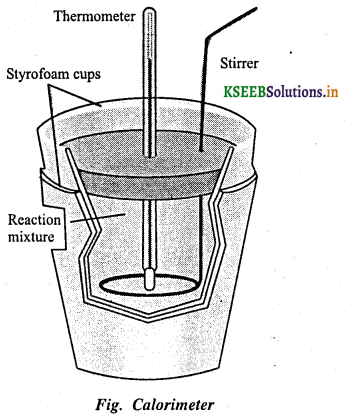Numerical Problem

Question 1.
1 g of graphite is burnt in a bomb calorimeter in excess of oxygen at 298 K and 1 atmospheric pressure according to the equation C(graphite) + O2(g) → CO2(g). During the reaction, temperature rises from 298 K to 299 K. If the heat capacity of the bomb calorimeter is 20.7 kJ/K, what is the enthalpy change for the above reaction at 298 K and 1 atm?
Quantity of heat absorbed by the calorimeter q = Cv × ΔT
Quantity of heat from the reaction will have the same magnitude but opposite sign because the heat lost by the system (reaction mixture) is equal to the heat gained by the calorimeter,
q = -Cv × ΔT
= -20.7 kJ/K × (299-298)K = -20.7 kJ
Thus, ΔU for the combustion of the 1 g of graphite = – 20.7 kJ K-1
ΔU for combination of 1 mol of graphite = $$\frac{12.0 \mathrm{g} \mathrm{mol}^{-1} \times(-20.7 \mathrm{kJ})}{1 \mathrm{g}}$$ = -2.48 × 102 kJ mol-1
Since, Δn = 0, ΔU = ΔH = -2.48 × 102 kJ mol-1

Enthalpy Change of Reaction

Question 1.
Define enthalpy of reaction. Illustrate with an example.
It is defined as “the change in enthalpy when all the moles of the reactants as indicated in the balanced chemical equation have completely reacted to form products.”
ΔH = ΣHproducts – ΣHreactant
Consider a reaction,
CH4 (g) + 2O2 (g) → CO2 (g) + 2H2O(1)
ΔH = [HC02 + 2HH2O] – [HCH4 + 2HO2]

Question 2.
Define standard enthalpy of reaction.
Ans:
The standard enthalpy of reaction is the enthalpy change for a reaction when all the participating substances are in their standard states.
The standard state of a substance at a specified temperature is its pure form at 1 bar. Usually data are taken at 298 K.

Question 3.
Define standard molar enthalpy of fusion. Give an example.
The enthalpy change that accompanies melting of one mole of a solid substance in standard state is called standard enthalpy of fusion or molar enthalpy of fusion.
Example, when 1 mol of ice is melted, we find from experiment that 6.01 kJ of enthalpy are needed at 273K. Thus, the standard molar enthalpy of fusion of ice is + 6.01 kJ mol-1 and we can write,
H2O(S) → H2O(1) ΔfusH° = + 6.01 kJ mol-1
Note: Melting of a solid is endothermic, so all enthalpies of fusion are positive.

Question 4.
Define standard molar enthalpy of vapourisation. Give an example.
The Amount of heat required to vapourise one mole of a liquid at constant temperature and under standard pressure (1 bar) is called its standard enthalpy of vapourisation or molar enthalpy of vapourisation. Example, standard molar enthalpy of vapourisation of water is + 40.79 kJ mol-1.
H2O(1) → H2O(g) ΔvapH° = + 40.79 kJ mol-1

Question 5.
Define standard enthalpy of sublimation. Give an example.
Sublimation is a process in which a solid substance directly changes into its vapours at any temperature below its melting point.
The change in enthalpy when one mole of a solid substance sublimes at a constant temperature and under standard pressure (1 bar) is called standard enthalpy of sublimation. For example, when one mole of solid iodine is converted into its vapours at room temperature, 62.4 kJ is absorbed. So, the enthalpy of sublimation of iodine is + 62.4 kJ mol-1,
I2(s) → I2(g) ΔHsub = +62.4 kJ mol-1
The magnitude of the enthalpy change depends on the strength of the intermolecular interactions in the substance undergoing the phase transformations. For example, the strong hydrogen bonds between water molecules hold them tightly in liquid phase. For an organic liquid, such as acetone, the intermolecular dipole-dipole interactions are significantly weaker. Thus, it requires less heat to vaporize 1 mol of acetone than it does to vaporize 1 mole of water.Question 6.
Define standard enthalpy of formation. Illustrate with an example.
It is the change in enthalpy produced when one mole of a compound is formed from its elements in their standard states.
Examples:
H2 (g) + $$\frac { 1 }{ 2 }$$O2 (g) → H2O (1) ΔH = -285.8KJ
Therefore, enthalpy of formation of water is – 285.8kJ
N2(g) + O2(g) → 2NO(g); ΔH = +180.7 KJ
Therefore, enthalpy of formation of nitric oxide is = +$$\frac{180 \cdot 7}{2}$$ = + 90.35KJ

Note .

• By using the standard enthalpy of formation values, we can calculate the enthalpy of reaction by the relation.
ΔrH° = ΣΔfproducts – ΣΔfreactints
Standard enthalpy change ΔrH° is expressed in kJ mol-1.
• The contribution of each compound is found by multiplying the heat of formation in kJ per mole by the number of moles of compound, given by its coefficient in the balanced equation.
• Standard enthalpy of formation of any element in its stable form is zero.
• Arbitrarily ΔfH° of H+ ion is assigned to be zero.
• Enthalpy of formation of a compound itself is the enthalpy of that compound.
• Standard enthalpies of formation of substances are inversely proportional to their stability.

Question 7.
ΔH°t of Fe2O3 is -825 kJ. What is the enthalpy change for the reaction?
4Fe2O3 → 8Fe + 6O2
ΔH = + 825 × 4 = 3300 kJ

Question 8.
The standard enthalpies formation of three substances A, B and C are 201.6, – 52.6 and – 106 kJ respectively. Arrange them in order of decreasing stability with respect to decomposition.
C > B > A

Question 9.
What is thermochemical equation? Give an example.
A balanced equation which shows liberation or absorption of heat and also physical states of reactants and products is called thermochemical equation. Example,
2H2(g) + O2(g) → 2H2O(g) ΔH = -483.6 kJ

Question 10.
State and illustrate Hess’s law of heat summation.
Hess’s law states that “the enthalpy change is same whether a reaction is carried out in one step or several steps”.
ΔH = ΔH1 + ΔH2 + ΔH3 + ΔH4 + ………..
Illustration of Hess’s Law: When carbon is burnt in excess of oxygen, carbon dioxide is formed and 393.5 kJ of heat is released per mole of carbon.
C(s) + O2(g) → CO2(g) ΔH = -393.5 kJ
The above reaction can also be carried out in two stages.
Carbon burns in limited oxygen producing carbon monoxide.
C(s) + $$\frac { 1 }{ 2 }$$O2 (g) → CO(g) ΔH = – 110.5 kJ

Carbon monoxide then burns in additional oxygen forming carbon dioxide.
CO(g) + $$\frac { 1 }{ 2 }$$O2 (g) → CO2(g) ΔH = – 283.0 kJ

These two equations can be added to calculate ΔH for the overall reaction:‘ΔH’ for this reaction producing carbon dioxide gas from solid carbon and oxygen is the same whether the reaction is done in one step or several steps.Enthalpies of Different Kinds of Reactions

Question 1.
Define standard enthalpy of combustion. Illustrate with an example.
It is the amount of heat liberated when one mole of a substance is burnt in excess of oxygen or air.
Example,
(1) The enthalpy of combustion of butane (cylinder gas) at 298 K is – 2658 kJ.
C4H10(g) + 13/2 O2(g) → 4CO2 (g) + 5H2O(g) ΔCH° = – 2658 kJ / mol

(2) The enthalpy of combustion of glucose at 298 K is – 2802kJ.
C6H12O6(g) + 6O2(g) → 6CO2 (g) + 6H2O(g) ΔCH° = – 2802 kJ/mol

Question 2.
What is enthalpy of atomization? Give examples.
The enthalpy change accompanying the dissociation of 1 mole of the given substance into gaseous atoms is called enthalpy of atomisation. This is often represented by the symbol AaH°.
Examples
(1) H2(g) → 2H(g); ΔaH° = 435.0 kJ mol-1
In case of diatomic molecules, like dihydrogen (given above), the enthalpy of atomization is also the bond dissociation enthalpy.
(2) CH4(g) → C(g) + 4H(g); ΔaH° = 1665 kJ mol-1
Note that the products are only atoms of C and H in gaseous phase
(3) Na(s) → Na(g); ΔaH° = 108.4 kJ mol-1 .

Question 3.
Discuss bond enthalpy by taking diatomic and poly atomic molecules.
Chemical reactions involve the breaking and making of bonds. Energy has to be supplied for the breaking of bonds and energy is released when bond is formed. There are two different terms used to explain bond enthalpy.

• Bond dissociation energy
• Mean bond enthalpy

ΔHrxn = Σ [ΔH(bonds broken)] – Σ[ΔH(bonds formed)]

The bond dissociation enthalpy is the change in enthalpy when one mole of covalent bonds of a gaseous covalent compound is broken to form atoms in the gas phase.

Ibis is a definite quantity and is expressed in kJ mol-1. For diatomic molecules the bond dissociation energy is same as bond enthalpy, whereas in polyatomic molecules the bond enthalpy is taken as the mean average of the various bond dissociation energies of the bonds of a given type.

Diatomic molecules:
Consider the following process in which the bonds in one mole of dihydrogen gas (H2) are broken:
H2(g) → 2H(g); ΔH-HH° = 435.0 kJ mol-1
The enthalpy change involved in this process is the bond dissociation enthalpy of H-H bond. Note that it is the same as the enthalpy of atomization of dihydrogen. This is true for all diatomic molecules.
For example:
Cl2(g) → 2Cl(g); Δc-clH° = 242 kJ mol-1
O2(g) → 2O(g); Δ0=0H° = 428 kJ mol-1

Polyatomic molecules:
Bond dissociation enthalpy is different for different bonds within the same molecule. The thermochemical data is useful in determining the bond energies of different bonds.
Let us consider a polyatomic molecule like methane, CH4. The overall thermochemical equation for its atomization reaction is CH4(g) → C(g) + 4H(g); ΔaH° = 1665 kJ mol-1
In methane, all the four C – H bonds are identical in bond length and energy. However, the energies required to break the individual C – H bonds in each successive step is differ.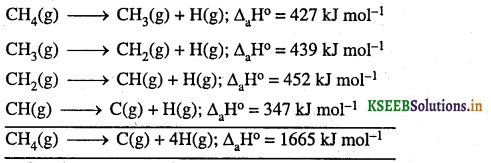In such cases we use mean bond enthalpy of C – H bond.
The mean bond enthalpy of C-H bond in methane is
ΔC-HH° in methane = $$\frac { 1 }{ 4 }$$(ΔaH° ) = $$\frac { 1 }{ 4 }$$ × 1665 = 416 kJ mol-1

Question 4.
Define enthalpy of solution. How do you calculate enthalpy of solution of an ionic compound?
Enthalpy of solution is defined as the heat change which occurs when one mole of a solute is dissolved in a large quantity of solvent such that further dilution does not cause further heat change.
For example, dissolution of sodium chloride
NaCl(s) + aq → NaCl(aq) ΔH = ΔHsol = +5.0 kJ
Here ‘aq’ represents aqueous meaning a large excess of water.
Enthalpy of solution is the sum of lattice enthalpy and enthalpy of hydration of ions in the solution.
ΔsolH° = ΔlatliceH° + ΔhydQuestion 5.
What is lattice enthalpy? How do you calculate the lattice enthalpy of sodium chloride by using Born- Haber cycle?
Lattice energy is defined as amount of energy required to separate one mole of solid ionic compound into its gaseous ions.
Calculation of lattice energy of sodium chloride by using Born-Haber cycleΔlatticeH = ΔsubH + ΔiH + ΔdissH + ΔegH – Δf
= 108 + 496 + 122 + (-348.6)-(-411.2)
ΔlatticeH = 788 kJ

Numerical Problems

Question 1.
The combustion of one mole of benzene takes place at 298 K and 1 atm. After combustion, CO2 (g) and H2O (l) are produced and 3267.0 kJ of heat is liberated. Calculate the standard enthalpy of formation (ΔfH°) of benzene. Standard enthalpies of formation of CO2(g) and H2O(l) are -393.5 kJ mol-1 and – 285.83 kJ mol-1 respectively.
The enthalpy of formation of benzene (required equation) is given by
6C(graphite) + 3H2(g) → C6H6(l) ΔfH° = ?

The enthalpy of combustion of 1 mole of benzene is:
C6H6(l) + $$\frac { 15 }{ 2 }$$O2(g) → 6CO2(g) + 3H2O(l); ΔcH° = -3267 KJ mol-1 ….. (1)

The enthaply of formation of 1 mol of CO2 (g);
C(graphite) + O2 (g) → CO2 (g) ΔfH° = -393.5 kj mol-1 …… (2)

The enthalpy of formation of 1 mole of H20(l) is
H2(g) + $$\frac { 1 }{ 2 }$$O2(g) → H2O(1) ΔfH° = -285.83 kJ mole-1 …… (3)

Applying Hess’s law
Equation (2) × 6 + (3) × 3 + Reverse equation (1), we get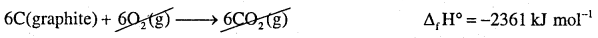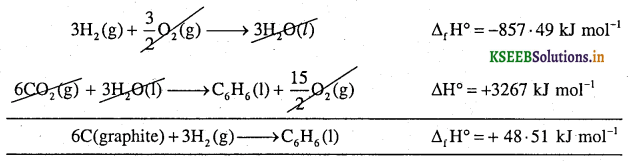Question 2.
Calculate the enthalpy of combustion of liquid benzene given that enthalpy of formation of benzene, CO2 and H2O are 48.51 kJ, – 393.5 kJ and – 285.83 kJ respectively.
The enthalpy of combustion of benzene (required equation) is given by
C6H6(l) + $$\frac { 15 }{ 2 }$$O2(g) → 6CO2(g) + 3H2O(1) ΔfH° = ?

The enthalpy of formation of benzene is given by
6C(g) + 3H2(g) → C6H6(1) ΔH° = +48.51 kJ mol-1 … (1)

The enthalpy of formation of carbon dioxide is given by
C(s) + O2(g) → CO2(g) ΔH° = -393.5kJ ….. (2)

The enthalpy of formation of water is given by
H2(g) + $$\frac { 1 }{ 2 }$$O2(g) → H2O(1) ΔH° = -285.83 kJ …(3)
Applying Hess’s law of heat summation
Equation (2) × 6 + (3) × 3 + Reverse equation (1) we getQuestion 3.
Calculate the enthalpy of formation of methane from the following data
C(s) + O2(g) → CO2(g) ΔH° = -393-5 kJ …(1)
H2(g) + $$\frac { 1 }{ 2 }$$O2(g) → H2O(1) ΔH° = -285.87 kJ …(2)
CH4(g) + 2O2(g) → CO2(g) + 2H2O(l) ΔH° = -890 kJ …..(3)
Enthalpy of formation of methane (required equation) is given by
C(s) + 2H2(g) → CH4(g) ΔfH = ?
Applying Hess’s law
Equation (1) × 1 + (2) × 2 + Reverse equation (3), we get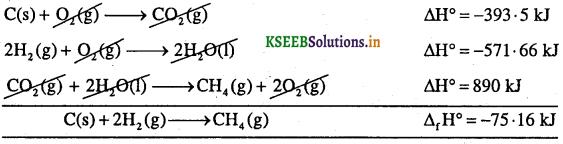Question 4.
Calculate the standard enthalpy of combustion of methane, Given that standard enthalpy of combustion of carbon and hydrogen are – 393.5 kJ and – 285.83 kJ respectively. Standard enthalpy of formation of methane is – 75.16 kJ.
The standard enthalpy of combustion (required equation) of methane is given by
CH4(g) + 2O2(g) → CO2(g) + 2H2O(l) ΔCH° = ?

The standard enthalpy of combustion of carbon is given by
C(s) + O2 (g) → CO2(g) ΔCH° = -393.5 KJ ……. (1)

The standard enthalpy of combustion of hydrogen is given by
H2(g) + $$\frac { 1 }{ 2 }$$O2(g) → H2O(1) ΔCH° = -285.83 KJ …… (2)

The standard enthalpy of formation of methane given by
C(s) + 2H2(g) → CH4(g) ΔfH° = -75 . 16 KJ ……. (3)

Applying Hess’s law;
Equation (1) × 1 + (2) × 2 + Reverse equation (3), add, we get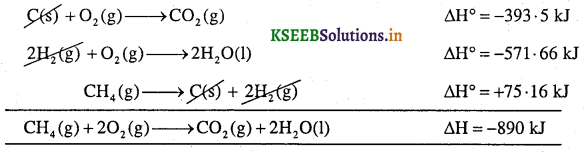Question 5.
Calculate the enthalpy of combustion of methyl alcohol (CH3OH) from the following given data.
Δf(H2O) = -285.83 KJ mol-1 Δf (CO2) = – 393.5 KJ mol-1 and ΔfH°(CH3OH) = -238 . 86 KJ mol-1
Enthalpy of combustion of methyl alcohol is given by
CH3OH(l) + $$\frac { 3 }{ 2 }$$ O2(g) → CO2(g) + H2O(l) ΔCH° = ?

The standard enthalpy of formation of H2O is given by
H2(g) + $$\frac { 1 }{ 2 }$$O2(g) → H2O(1) ΔH = -285.83 kJ mol-1 …(1)

The standard enthalpy of formation of CO2 is given by
C(s) + O2(g) → CO2(g) ΔH = -393.5 kJ mol-1 …(2)

The standard enthalpy of formation of methyl alcohol is given by
C(s) + 2H2(g) + $$\frac { 1 }{ 2 }$$ O2(g) → CH3OH(l) ΔH = -238.86 kJ mol-1 …(3)
Applying Hess’s law
Equation (1) × 2 + (2) × 1 + Reverse equation (3), we get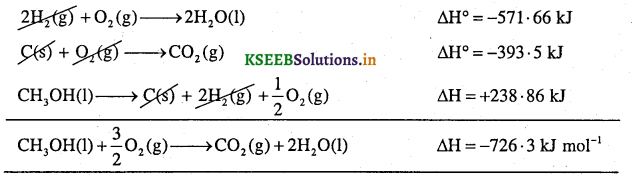Question 6.
Calculate the standard enthalpy of formation of methanol. Given that enthalpy of combustion of carbon, hydrogen and methanol are – 393.5 kJ k mol-1, – 285.83 kJ mol-1 and – 726.3 kJ mol-1 respectively.
The standard enthalpy of formation of methanol (required equation) is given by
C(s) + 2H2(g) + $$\frac { 1 }{ 2 }$$O2(g) → CH3OH(l) ΔfH° = ?

Given, the standard enthalpy of combustion of carbon is given by
C(s) + O2(g) → CO2(g) ΔCH° = -393. 5 kJmol-1 …(1)

The standard enthalpy of combustion of hydrogen is given by
H2(g) + $$\frac { 1 }{ 2 }$$ O2(g) → H2O(1) ΔCH° = -285.83 kJ mol-1 …(2)

The standard enthalpy of combustion of methanol is given by
CH3OH(l) + $$\frac { 3 }{ 2 }$$ O2(g) → CO2(g) + 2H2O(l) ΔCH° = -726.3kJ …(3)

Applying Hess’s law:
Equation (1) × 1 + (2) × 2 + reverse equation (3), we getSpontaneity

Question 1.
What is spontaneous process? Give an example.
A process (physical or chemical) which occurs on its own with or without proper initiation under the given set of conditions is called spontaneous process.
1. Examples for chemical spontaneous processes
a) Combination of ammonia and hydrogen chloride giving dense white fumes of ammonium chloride.
HCl(g) + NH3 (g) → NH4Cl(S)
b) Oxidation of nitric oxide to nitrogen dioxide by air.
2NO(g) + O2 (g) → 2NO2(g)

2. Examples for physical process
a) Flow of water from a higher level to a lower level.
b) Flow of heat from hot end to cold end.
c) Evaporation of water.

3. Examples of spontaneous processes, which require initiation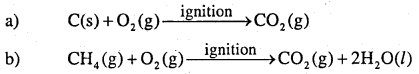Note: A spontaneous process is an irreversible process and can only be reversed with the aid of some external agency.

Question 2.
Decrease in enthalpy is a criterion for spontaneity. Explain by taking enthalpy diagrams.
Every system tends to attain a state of minimum energy and maximum stability.
Thermodynamically, energy change in chemical reaction is expressed in terms of its enthalpy change (ΔH):
ΔH = Hp – HR
Enthalpy level diagrams can be used to illustrate overall exothermic and endothermic changes. They show the difference in total enthalpy of the reactants and products for a reaction.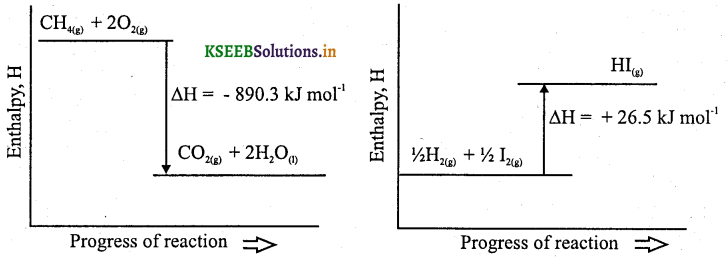For an exothermic reaction the total enthalpy of the products is less than that of reactants. For an endothermic reaction the total enthalpy of the reactants is less than that of the products. In each case, the difference in these total enthalpies is equal to the overall enthalpy of the reaction, ΔH. Most of the spontaneous reactions are exothermic. In exothermic reactions, Hp < HR, i.e., ΔH = -ve. Hence, negative value of ΔH (decrease in enthalpy change) is one criterian for the spontaneity of a process. However, negative ΔH or decrease in energy is not the sole criterion for spontaneity.Question 3.
What is entropy?
Entropy is a measure of disorder mass or randomness of a system.
In a reversible process, entropy is calculated by As = $$\frac{\mathrm{q}_{\mathrm{rev}}}{\mathrm{T}}$$

Note:
Change in entropy of a reaction can be calculated from the entropy values of the reactants and products as
Entropy change in a reversible process is zero.
Entropy change in irreversible process is always positive (greater than zero).
Entropy of phase transition.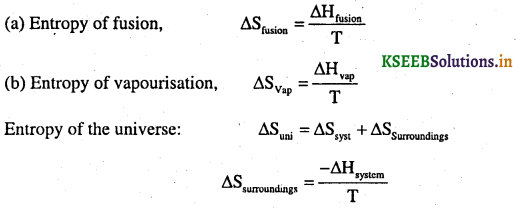Question 4.
For oxidation of iron, 4Fe(s) + 3O2(g) → 2Fe2O3(s) entropy change is -549.4 JK-1 mol-1< at 298 K. Inspite the negative entropy change of this reaction, why is the reaction spontaneous? ( ΔrH° for this reaction is -1684 × 105 J mol-1)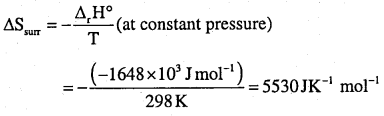Thus, total entropy change for the reaction
ΔStotal = ΔSsystem + ΔSsurrounding
ΔStotal = 5530 + (-549.4) = 4980.6 JK-1 mol-1 (highly positive value)
This shows that the above reaction is spontaneous.

Question 5.
Predict in which of the following, entropy increases/decreases:
(i) A liquid crystallizes into a solid.
(ii) Temperature of a crystalline solid is raised from 0 K to 115 K
(iii) 2NaHCO3(s) → Na2CO3(s) + CO2(g) + H2O(g)
(iv) H2(g) → 2H(g)
(i) After freezing, the molecules attain an ordered state and therefore, entropy decreases.

(ii) At 0 K, the constituent particles are static and entropy is minimum. If temperature is raised to 115 K, these begin to move and oscillate about their equilibrium positions in the lattice and the system becomes more disordered, therefore entropy increases.

(iii) Reactant, NaHCO3 is a solid and it has low entropy. Among products there are one solid and two gases. Therefore, the products represent a condition of higher entropy.

(iv) Here one molecule gives two atoms i.e., number of particles increases leading to more disordered state. Two moles of H atoms have higher entropy than one mole of dihydrogen molecule.Question 6.
Define Gibbs free energy (G)
The portion of enthalpy of a system that is available for useful work is called Gibb’s free energy. It is expressed as
G = H – TS.

Question 7.
Write Gibb’s equation.
ΔG = ΔH – TΔS

Question 8.
Give the significance of AG.
a) When ΔG = -ve(ΔG < O), the process is spontaneous.
b) When ΔG = +ve(ΔG > O), the process is non-spontaneous.
c) When ΔG = O, the process is at equilibrium.
Note: Decrease in free energy is the main criterian for all spontaneous processes.

Question 9.
Discuss the effect of temperature on spontaneity of reactions.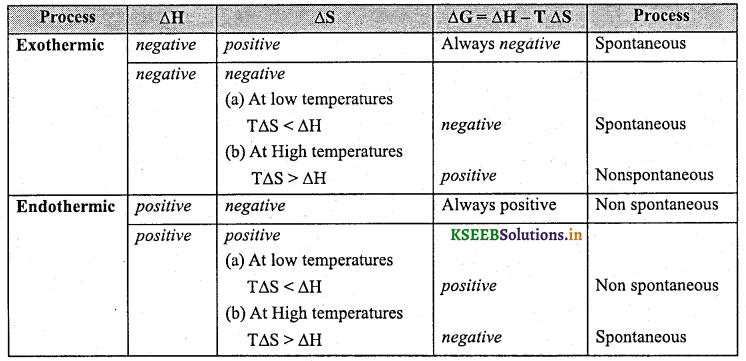Question 10.
What are the conditions of ΔH and ΔS for a reaction to be spontaneous at any temperature?
ΔH = – ve, ΔS = + ve.

Question 11.
Write the relation between Gibbs free energy change and equilibrium constant.
ΔG° = -2.303 RT log K
where ΔG° → standard free energy change.
R → universal constant
T → Temperature in K
K – Equilibrium constant
Note: In spontaneous reaction ΔG° <0 if K > 1
In non spontaneous reaction ΔG° >0 if K < 1

Question 12.
For the equilibrium A + 2B ⇌ C, ΔH is + 400 KJ and ΔS is + 200 JK-1 Calculate the temperature above which the reaction becomes spontaneous.
ΔG = ΔH – TΔS
At equilibrium, ΔG = 0
O = ΔH – TDS
T = $$\frac{\Delta \mathrm{H}}{\Delta \mathrm{S}}=\frac{400 \times 1000}{200}$$ = 2000 k.

Question 13.
ΔH and ΔS for a certain chemical reaction at 298 K are – 1684 × 103 J mol-1 and – 549.4 JK-1 mol-1 Calculate ΔG for the reaction. Is the reaction spontaneous?
ΔG = ΔH-TΔS
=-1684 × 1000 – 298 × (-549.4)
= -1684000 + 163721.2
= -1520278.8 J
= -1520.27 kJ
Since ΔG = -ve, the reaction is spontaneous.Question 14.
The value of standard free energy of formation of ammonia at 298 K is -16.6 kJ mol-1. Calculate the equilibrium constant Kp for the reaction.
ΔG° = -2.303RTlog10 Kp
-16.6 × 103 = -2.303 × 8.314 × 2981og10Kp
1og10Kp = $$\frac{16.6 \times 10^{3}}{2.303 \times 8.314 \times 298}$$
Kp = 2.9092
Kp = Anti log (2-9092)
Kp = 8.114 × 102

Question 15.
The partial pressure equilibrium constant for a reaction is 7.5xl03 at 298 K. Calculate the standard free energy change.
ΔG° =-2.303RT log10 Kp
= -2.303 × 8.314 × 298 log7.5 × 103 = -2.303 × 8.314 × 298 × 3.8751 = -22110.73 J mol-1

Question 16.
The standard free energy change of a reaction is 100 kj. Calculate Kp.
ΔG° = -2.303RT log10 Kp
100 × 103 = -2.303 × 8.314 × 298 × log Kp
log Kp = $$-\frac{100 \times 1000}{2.303 \times 8.314 \times 298}$$
log Kp = -17.52
Kp = Antilog (-17.52)
= Antilog $$(\overline{18} \cdot 48)$$
Kp = 3.02 × 10-18

Question 17.
Calculate ΔrG° for conversion of oxygen to ozone, 3/2O2(g) → O3(g) at 298 K, if Kp for this conversion is 2.47 × 10-29. [NCERT example]
ΔrG° = -2.303RT logKp
ΔrG° = -2.303 × (8.314 JK-1 mol-1) × (298 K) (log 2.47 × 10-29)
= 163000J mol-1 = 163 kJ mol-1

Question 18.
Find out the value of equilibrium constant for the following reaction at 298 K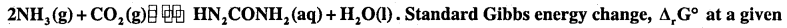temperature is – 13.6 kJ mol-1.
ΔG° = -2.303 RT log Kp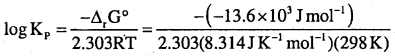Hence Kp = antilog (2.3835)
= 5.841 × 102Question 19.
At 60°C, dinitrogen tetroxide is fifty percent dissociated. Calculate the standard free energy change at this temperature and at one atmosphere.If N2O4 is 50% dissociated, the mole fraction of both the substances is given by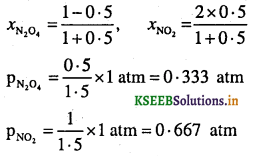The equilibrium constant Kp is given by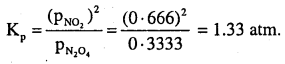Since ΔrG° = -2 . 303 RT log Kp
ΔrG° =(-8.314 JK-1 mol-1) × (333 K) × 2.303 × log 1.3333.
ΔrG° =(-8-314 JK-1 mol-1) × (333 K) × 2.303 × 0.1239 .
= -763.8 kJ mol-1

Question 20.
State second law of thermodynamics.